Wacker Art Basic FunctionsBild: "Kellerwald"

## Mathematical Functions - Basic Functions

### Taylor Series of the Exponential Function### Even Part of the Exponential Function - The Hyperbolic CosineA function is called even if f(x) = f(-x)

### Odd Part of the Exponential Function - The Hyperbolic Sine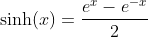A function is called odd if f(x) = -f(-x)

### Taylor Series of the Hyperbolic Cosine Function### Taylor Series of the Hyperbolic Sine Function### Relation between Hyperbolic Cosine Function and Cosine Function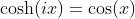### Relation between Hyperbolic Sine Function and Sine Function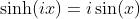### Taylor Series of the Cosine Function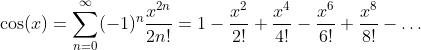### Taylor Series of the Sine Function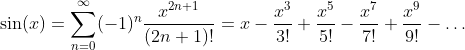### Basic Relations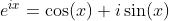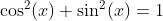### Definition of Sine and Cosine Functions with the Complex Exponential Function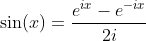### Definition of Tangent and Cotangent Functions with Sine and Cosine Functions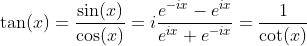### Hyperbolic Tangent and Hyperbolic Cotangent Functions### Logarithm Function: y = ln(x)

The logarithm function is defined for x values in the range 0 < x < ∞.

### Taylor Series

Radius of convergence: |x| < 1 and x = 1.### Definition with arctanh### Integral of ln(x)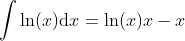### Derivative of ln(x)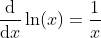### Further Properties of ln(x)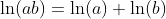### Logarithm Functions loga(x) with Different Bases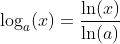### Logarithm loga(x) with Different Bases a

 a = 1/2 a = 2 a = e a = 10

### Integral of ln(x)### Function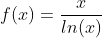This function can be used for the approximation of the prime counting function π(x).This relation is also known as prime number theorem.

### Special Function### Derivative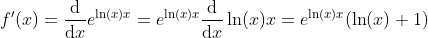### Taylor Series### Logistic Function: y = 1/(1 + e-x)

Formulars of the logistic function: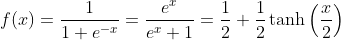The logistic function is the solution of the logistic differential equation.

### Integration of the Logistic Function

Using the substitution u = 1 + ex and u' = ex gives du = exdx.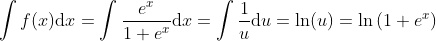### Derivative of the Logistic Function### Bernoulli Function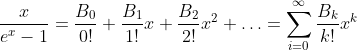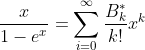The Bi are the Bernoulli Numbers.

### Bernoulli Numbers

The Bernoulli numbers Bi and Bernoulli numbers Bi* differ only in the value of i= 1. B1=-1/2 and B1* = 1/2, where they only have different sign. All the other values are the same.

 i 0 1 2 3 4 5 6 7 8 9 10 11 12 ... Bi 1 ±1/2 1/6 0 -1/30 0 1/42 0 -1/30 0 5/66 0 -291/2730 ...

### Taylor Series### Relations### Inverse Hyperbolic Sine Function - Area Sinus Hyperbolicus: y = arsinh(x)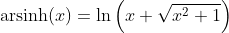### Taylor Series### Relations### Invers Hyperbolic Cosine Function - Area Cosinus Hyperbolicus: y = arcosh(x)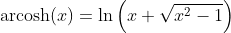### Hyperbolic Tangent Function - Tangens Hyperbolicus: y = tanh(x)### Inverse Hyperbolic Tangent Function - Area Tangens Hyperbolicus: y = artanh(x)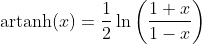### Hyperbolic Cotangent Function - Cotangens Hyperbolicus: y = coth(x)### Inverse Hyperbolic Cotangent Function - Area Cotangens Hyperbolicus: y = arcoth(x)### Sine Function: y = sin(x)### Inverse Sine Function - Arcus Sine: y = arcsin(x)### Cosine Function: y = cos(x)### Inverse Cosine Function - Arcus Cosine: y = arccos(x)### Tangent Function: y = tan(x)### Inverse Tangent Function: y = arctan(x)### Cotangent Function: y = cot(x)### Invers Cotangent Function: y = arccot(x)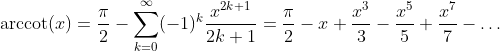### Cube Root Function: y = x1/3

Next Page:

06. März 2021 Version 2.0
Copyright: Hermann Wacker Uhlandstraße 10 D-85386 Eching bei Freising Germany Haftungsausschluß